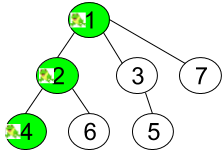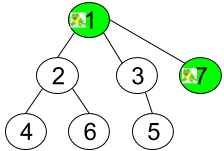Formatted question description: https://leetcode.ca/all/1377.html

# 1377. Frog Position After T Seconds (Hard)

Given an undirected tree consisting of n vertices numbered from 1 to n. A frog starts jumping from the vertex 1. In one second, the frog jumps from its current vertex to another unvisited vertex if they are directly connected. The frog can not jump back to a visited vertex. In case the frog can jump to several vertices it jumps randomly to one of them with the same probability, otherwise, when the frog can not jump to any unvisited vertex it jumps forever on the same vertex.

The edges of the undirected tree are given in the array edges, where edges[i] = [fromi, toi] means that exists an edge connecting directly the vertices fromi and toi.

Return the probability that after t seconds the frog is on the vertex target.

Example 1:Input: n = 7, edges = [[1,2],[1,3],[1,7],[2,4],[2,6],[3,5]], t = 2, target = 4
Output: 0.16666666666666666
Explanation: The figure above shows the given graph. The frog starts at vertex 1, jumping with 1/3 probability to the vertex 2 after second 1 and then jumping with 1/2 probability to vertex 4 after second 2. Thus the probability for the frog is on the vertex 4 after 2 seconds is 1/3 * 1/2 = 1/6 = 0.16666666666666666.


Example 2:Input: n = 7, edges = [[1,2],[1,3],[1,7],[2,4],[2,6],[3,5]], t = 1, target = 7
Output: 0.3333333333333333
Explanation: The figure above shows the given graph. The frog starts at vertex 1, jumping with 1/3 = 0.3333333333333333 probability to the vertex 7 after second 1.


Example 3:

Input: n = 7, edges = [[1,2],[1,3],[1,7],[2,4],[2,6],[3,5]], t = 20, target = 6
Output: 0.16666666666666666


Constraints:

• 1 <= n <= 100
• edges.length == n-1
• edges[i].length == 2
• 1 <= edges[i], edges[i] <= n
• 1 <= t <= 50
• 1 <= target <= n
• Answers within 10^-5 of the actual value will be accepted as correct.

Related Topics:
Depth-first Search

## Solution 1. DFS

Build the tree then try to generate the path from 1 to target.

• If t is not enough for reaching target, i.e. t + 1 < path.size(), then return 0
• If t is too long that the frog jumps further than the target, i.e. t + 1 > path.size() && tree[path.back()].size(), then return 0.
• Otherwise, the answer is 1.0 / k where k is the product of all the count of branches from the root to the parent of target.
// OJ: https://leetcode.com/problems/frog-position-after-t-seconds/

// Time: O(N)
// Space: O(N)
class Solution {
unordered_map<int, vector<int>> g, nei;
bool dfs(int start, int target, vector<int>&path) {
path.push_back(start);
if (start == target) return true;
for (int n : g[start]) {
if (dfs(n, target, path)) return true;
}
path.pop_back();
return false;
}
void buildTree(int start, int p) {
for (int n : nei[start]) {
if (n == p) continue;
g[start].push_back(n);
buildTree(n, start);
}
}
public:
double frogPosition(int n, vector<vector<int>>& edges, int t, int target) {
for (auto & e : edges) {
nei[e].push_back(e);
nei[e].push_back(e);
}
buildTree(1, -1);
vector<int> path;
dfs(1, target, path);
if (t + 1 < path.size() || (t + 1 > path.size() && g[path.back()].size())) return 0;
int cnt = 1;
for (int i = 0; i < path.size() - 1; ++i) cnt *= g[path[i]].size();
return 1. / cnt;
}
};


## Solution 2.

// OJ: https://leetcode.com/problems/frog-position-after-t-seconds/

// Time: O(N)
// Space: O(N)
// Ref: https://leetcode.com/problems/frog-position-after-t-seconds/discuss/532571/Python-DFS
class Solution {
vector<vector<int>> g;
vector<bool> seen;
double dfs(int node, int t, int target) {
seen[node] = true;
int cnt = g[node].size() - (node != 1);
if (!t) return node == target;
if (node == target) return !cnt;
double ans = 0;
for (int n : g[node]) {
if (seen[n]) continue;
if (ans = dfs(n, t - 1, target)) break;
}
if (ans) ans /= cnt;
return ans;
}
public:
double frogPosition(int n, vector<vector<int>>& edges, int t, int target) {
g.resize(n + 1);
seen.resize(n + 1);
for (auto &e : edges) {
g[e].push_back(e);
g[e].push_back(e);
}
return dfs(1, t, target);
}
};


Java

class Solution {
public double frogPosition(int n, int[][] edges, int t, int target) {
Map<Integer, Set<Integer>> edgesMap = new HashMap<Integer, Set<Integer>>();
for (int[] edge : edges) {
int vertex0 = edge - 1, vertex1 = edge - 1;
Set<Integer> next0 = edgesMap.getOrDefault(vertex0, new HashSet<Integer>());
edgesMap.put(vertex0, next0);
Set<Integer> next1 = edgesMap.getOrDefault(vertex1, new HashSet<Integer>());
edgesMap.put(vertex1, next1);
}
int[] parents = new int[n];
parents = -1;
boolean[] visited = new boolean[n];
visited = true;
bfsQueue.offer(0);
while (!bfsQueue.isEmpty()) {
int vertex = bfsQueue.poll();
Set<Integer> nextVertices = edgesMap.getOrDefault(vertex, new HashSet<Integer>());
for (int nextVertex : nextVertices) {
if (!visited[nextVertex]) {
visited[nextVertex] = true;
parents[nextVertex] = vertex;
bfsQueue.offer(nextVertex);
}
}
}
int targetPathLength = 0;
Set<Integer> targetPathSet = new HashSet<Integer>();
int curVertex = target - 1;
while (curVertex != 0) {
curVertex = parents[curVertex];
targetPathLength++;
}
if (targetPathLength > t)
return 0;
Map<Integer, Set<Integer>> childrenMap = new HashMap<Integer, Set<Integer>>();
for (int i = 0; i < n; i++) {
int parent = parents[i];
if (parent >= 0) {
Set<Integer> children = childrenMap.getOrDefault(parent, new HashSet<Integer>());
childrenMap.put(parent, children);
}
}
if (targetPathLength < t) {
Set<Integer> targetChildren = childrenMap.getOrDefault(target - 1, new HashSet<Integer>());
if (targetChildren.size() > 0)
return 0;
}
double probability = 1.0;
queue.offer(0);
while (!queue.isEmpty()) {
int parent = queue.poll();
Set<Integer> children = childrenMap.getOrDefault(parent, new HashSet<Integer>());
int childrenSize = children.size();
if (childrenSize == 0)
break;
probability /= childrenSize;
children.retainAll(targetPathSet);
for (int child : children) {
visited[child] = true;
queue.offer(child);
}
}
return probability;
}
}